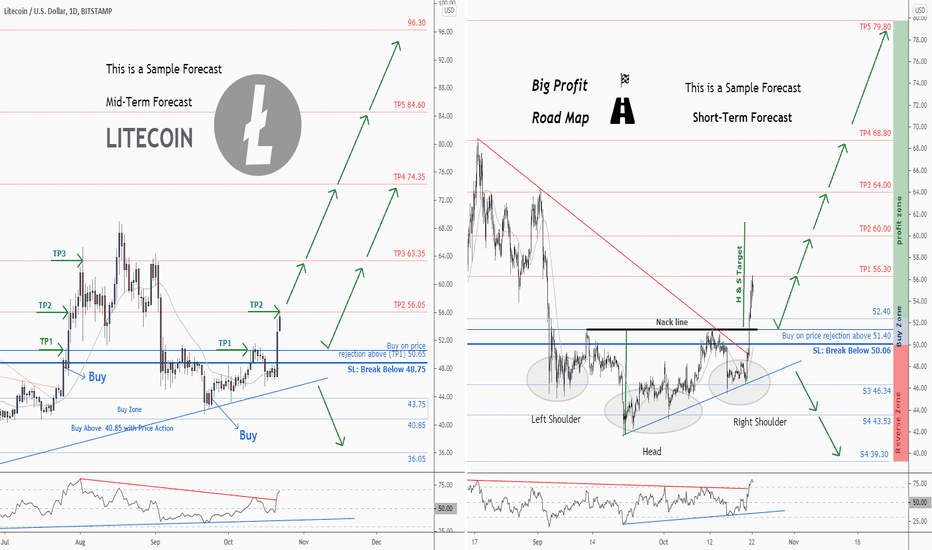DONATE & Share Post Your Content On Smart Money Management# 💡 Two buy opportunity in LTCUSD , Mid-Term & Short-Term

Litecoin / U.S. dollar BITSTAMP:LTCUSDShort-Term Forecast:

". There is a possibility of temporary retracement to suggested support line (51. 40 ).
. if so, traders can set orders based on Price Action and expect to reach short-term targets."

Technical analysis:
. LTCUSD is in a range bound and the beginning of uptrend is expected.
. The price is above the 21-Day WEMA which acts as a dynamic support.
. The RSI is at 76.

Take Profits:
TP1= @ 56.30
TP2= @ 60.00
TP3= @ 64.00
TP4= @ 68.80
TP5= @ 79.82

SL= Break below S2

--------------------------------------------------------------------------------------------------------
--------------------------------------------------------------------------------------------------------
--------------------------------------------------------------------------------------------------------

Mid-Term Forecast:

Profit targets=945% = {TP2= 10% + TP3= 25% + TP4= 46% + TP5= 67% + TP6= 106% + TP7= 134% + TP8= 189% + TP9= 368%}
Stop Loss = 36%
Reward/Risk > 26 : 1

". There is still a possibility of temporary retracement to suggested support line (50.65) again.
if so, traders can set orders based on Price Action and expect to reach short-term targets."

New Take Profits:
TP2= 56.05
TP3= 63.35
TP4= 74.35
TP5= 84.60
TP6= 104.45
TP7= 118.80
TP8= 146.55
TP9= 196.55
TP10= 237. 40
TP11= Free

SL= Break below 48.75

--------------------------------------------------------------------------------------------------

Total Profit: 275%

We opened 11 BUY trade(s) @ 43.75 based on 'previous Forecast' at 09.24.2020:

Closed Profit:
TP1 @ 50.65 touched at 10.11.2020 with 13% Profit.
TP2 @ 56.05 touched at 10.22.2020 with 28% Profit.
13% + 28% = 41%

Open Profit:
Open trade is 55.30 (current price) - 43.75 (open price) = 26%
9 trade(s) still open, therefore total profit for open trade(s) is 26% x 9 = 234%

Technical analysis:
. LTCUSD is in a range bound and the beginning of uptrend is expected.
. The price is above the 21-Day WEMA which acts as a dynamic support.
. The RSI is at 69.

❤️ If you find this helpful and want more FREE forecasts in TradingView

. . . . . . . . Hit the 👍 LIKE button,
. . . . . . . . . . . Drop some feedback below in the comment!

❤️ Your Support is very much 🙏 appreciated!❤️